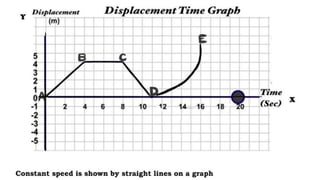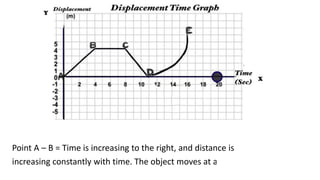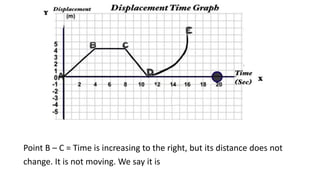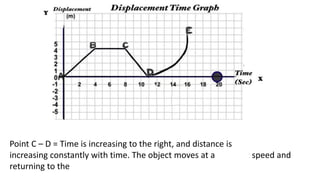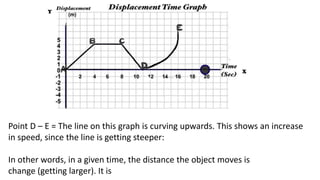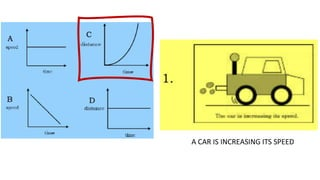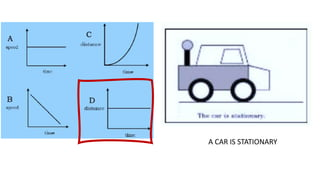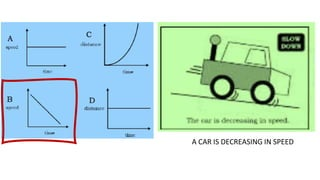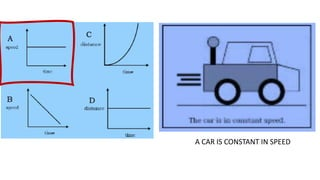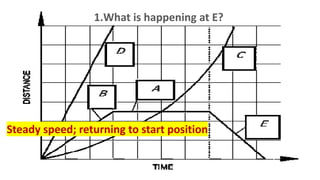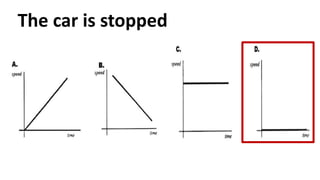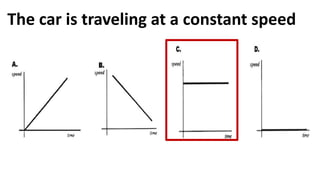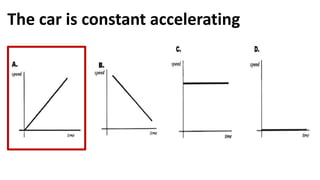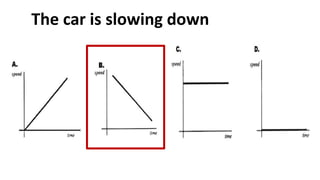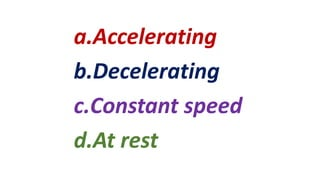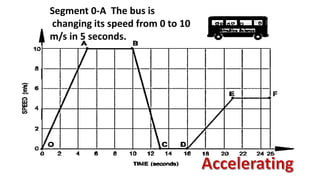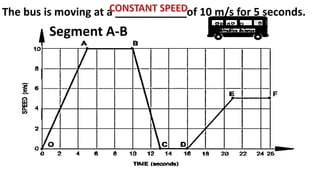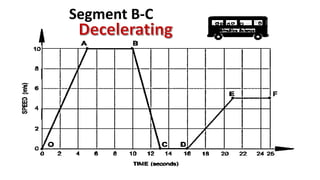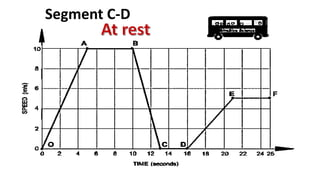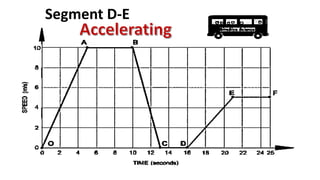1 de 22

### REVIEW.pptx

1. REVIEW MOTION IN GRAPHS
2. Point A – B = Time is increasing to the right, and distance is increasing constantly with time. The object moves at a constant speed.
3. Point B – C = Time is increasing to the right, but its distance does not change. It is not moving. We say it is At Rest.
4. Point C – D = Time is increasing to the right, and distance is increasing constantly with time. The object moves at a constant speed and returning to the initial position.
5. Point D – E = The line on this graph is curving upwards. This shows an increase in speed, since the line is getting steeper: In other words, in a given time, the distance the object moves is change (getting larger). It is accelerating.
6. A CAR IS INCREASING ITS SPEED
7. A CAR IS STATIONARY
8. A CAR IS DECREASING IN SPEED
9. A CAR IS CONSTANT IN SPEED
10. 1.What is happening at E? Steady speed; returning to start position
11. The car is stopped
12. The car is traveling at a constant speed
13. The car is constant accelerating
14. The car is slowing down
15. a.Accelerating b.Decelerating c.Constant speed d.At rest
16. Segment 0-A The bus is changing its speed from 0 to 10 m/s in 5 seconds.
17. The bus is moving at a ____________of 10 m/s for 5 seconds. Segment A-B
18. Segment B-C
19. Segment B-C
20. Segment C-D
21. Segment D-E